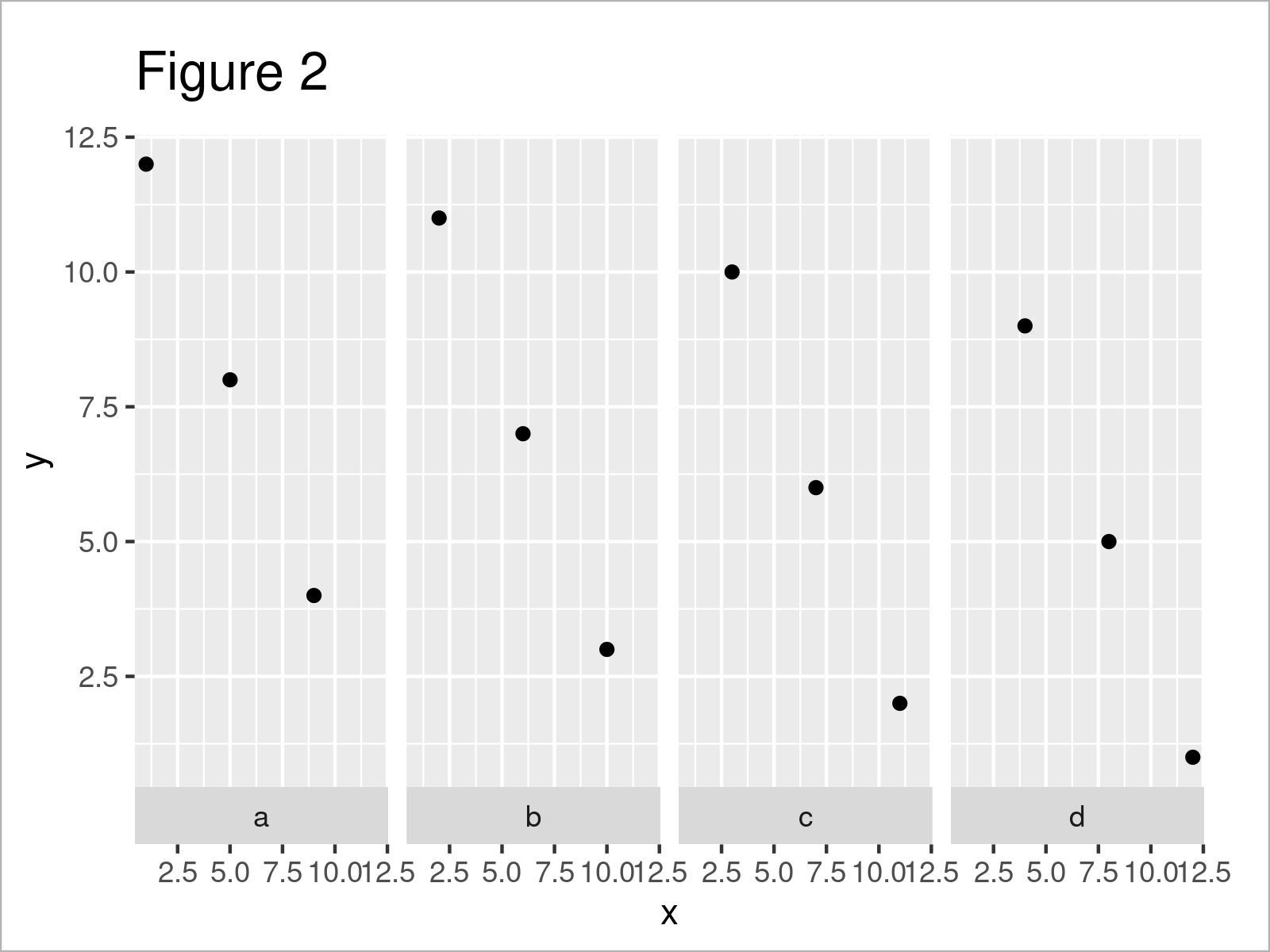# Move ggplot2 Facet Plot Labels to the Bottom in R (Example)

On this page, I’ll illustrate how to draw facet plot labels at the bottom of each panel in the R programming language.

Let’s dive right into the example…

## Example Data, Add-On Packages & Basic Graphic

Let’s first construct some example data:

```data <- data.frame(group = letters[1:4],    # Create example data
x = 1:12,
y = 12:1)
data                                        # Print example data```As you can see based on Table 1, our example data is a data frame and consists of twelve rows and three columns.

In order to use the functions of the ggplot2 package, we also have to install and load ggplot2:

```install.packages("ggplot2")                 # Install & load ggplot2
library("ggplot2")```

Now, we can draw our data as follows:

```ggplot(data, aes(x, y)) +                   # Draw default facet plot
geom_point() +
facet_grid(~ group)```In Figure 1 you can see that we have created a facet plot using the facet_grid function as shown in the previous R syntax.

The strip labels are shown at the top of each plot panel. In the following example, I’ll show how to change that!

## Example: Move Facet Plot Labels from Top to Bottom Using switch Argument

In this section, I’ll explain how to adjust the location of the facet plot labels so that the labels are shown below the plot.

For this task, we have to specify the switch function to be equal to “both” as shown in the following R code:

```ggplot(data, aes(x, y)) +                   # Move labels to bottom
geom_point() +
facet_grid(~ group, switch = "both")```In Figure 2 you can see that we have plotted a new version of our facet graph where the text labels are shown at the bottom.

## Video, Further Resources & Summary

Do you need further information on the contents of this article? Then you might want to watch the following video that I have published on my YouTube channel. I’m explaining the examples of this tutorial in the video.

Please accept YouTube cookies to play this video. By accepting you will be accessing content from YouTube, a service provided by an external third party.If you accept this notice, your choice will be saved and the page will refresh.

Furthermore, you may have a look at the other articles on this website.

This article has shown how to draw facet plot labels at the bottom position in the R programming language. Let me know in the comments, if you have further questions.

Subscribe to the Statistics Globe Newsletter